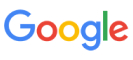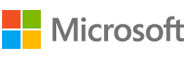New update is available. Click here to update.
Topics

# Farthest Distance From Lands

Moderate0/80
Average time to solve is 30m## Problem statement

You are given a binary square matrix ‘ARR’ with N rows and N columns, in which 0 represents the water and 1 represents the land.

You have to find a water cell such that its distance to the nearest land cell is maximized and print the maximum distance.

Note :

``````The distance between any two cells (x0, y0) and (x1, y1) is given by the Manhattan distance: |x0 - x1| + |y0 - y1|.
If no land or water exists in the grid, then return -1.
``````
Detailed explanation ( Input/output format, Notes, Images )
Constraints :
``````1 <= T <= 5
1 <= N <= 10^3
ARR[i][j] = 0 or 1

Time limit: 1 sec
``````
Sample Input 1 :
``````2
3
1 0 1
0 0 0
1 0 1
3
1 0 0
0 0 0
0 0 0
``````
Sample Output 1 :
``````2
4
``````
Explanation For Sample Input 1 :
``````For the first test case, The cell (1, 1) is as far as possible from all the land with distance 2.

For the second test case, The cell (2, 2) is as far as possible from all the land with distance 4.
``````
Sample Input 2 :
``````2
3
1 0 1
0 1 0
1 0 1
2
1 1
1 1
``````
Sample Output 2 :
``````1
-1
``````Console